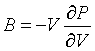## Tight binding

1-D crystal, one band

1-D crystal, two bands (trans-polyacetylene)

2-D square lattice

2-D hexagonal lattice

Graphene

2-D boron nitride

Carbon nanotubes

simple cubic 3-D

fcc

bcc

hexagonal

Dispersion relation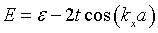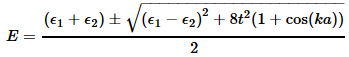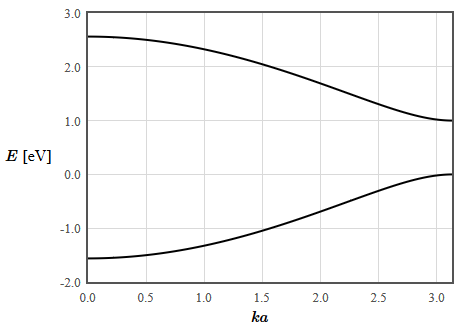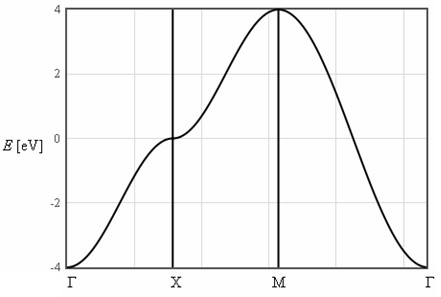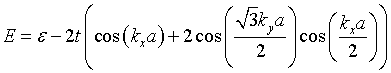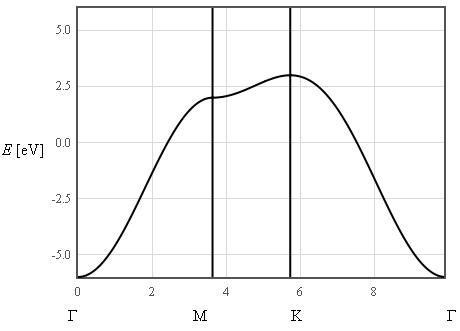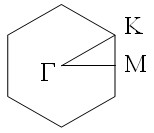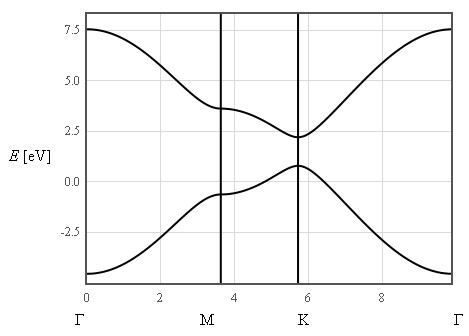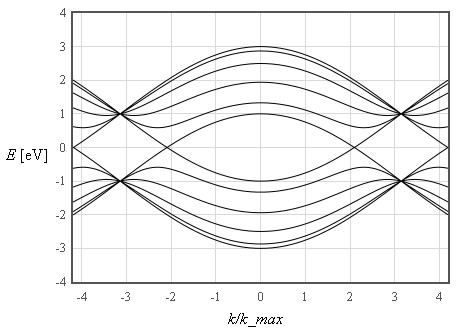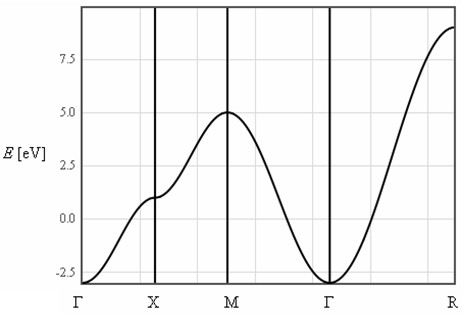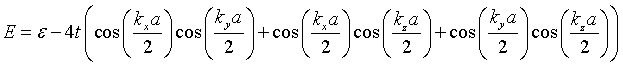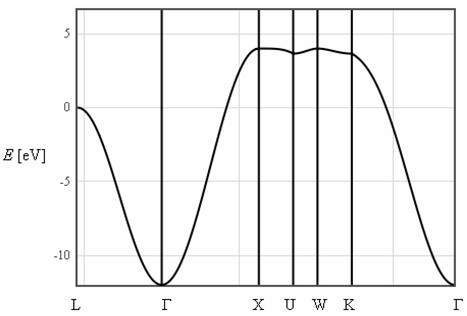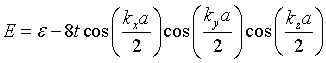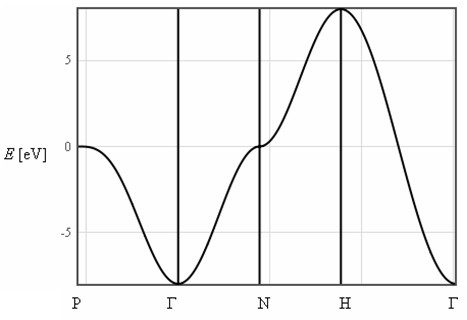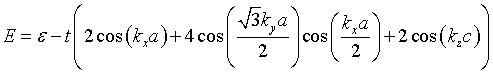Density of states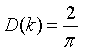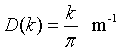Density of states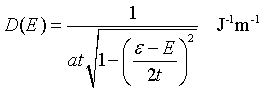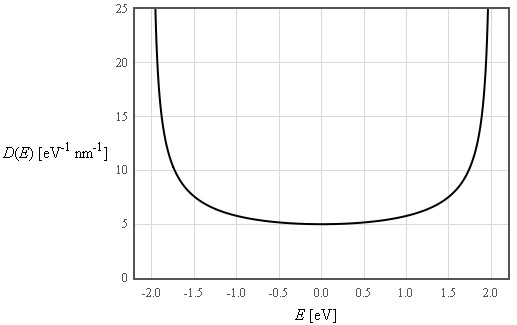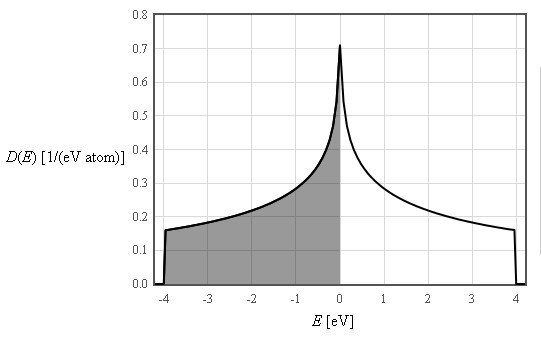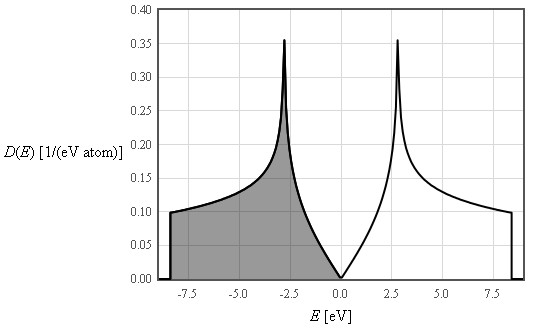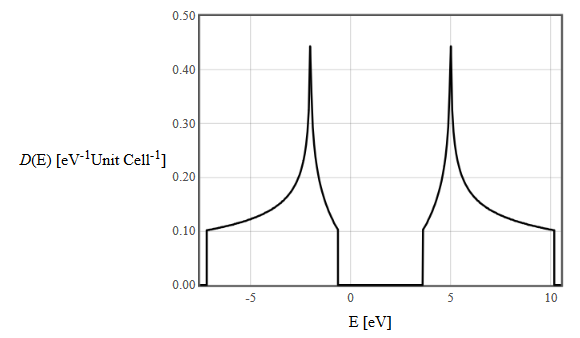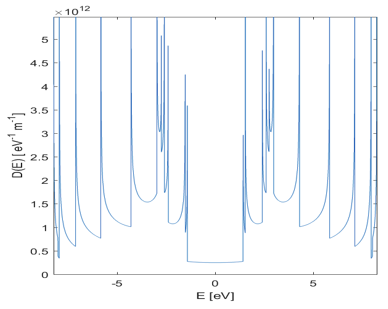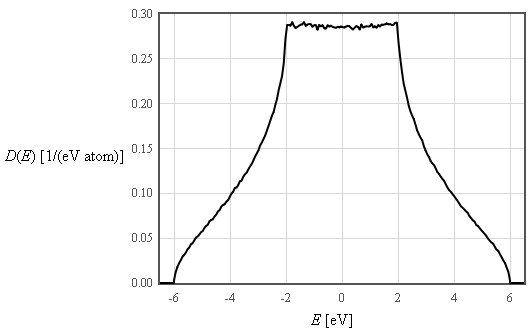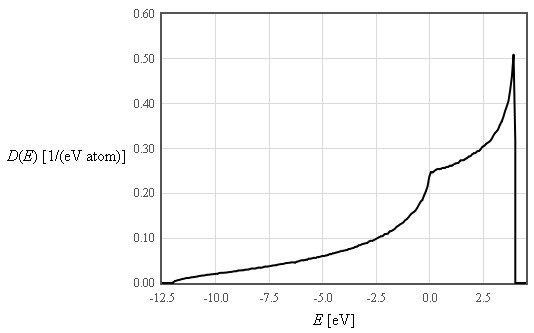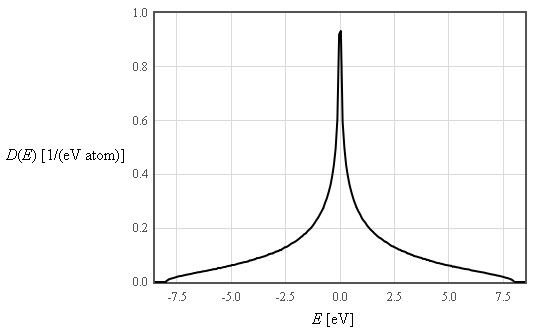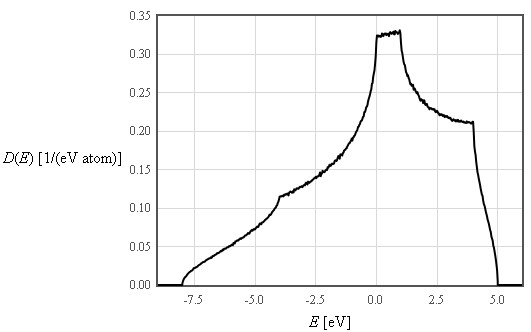Fermi surface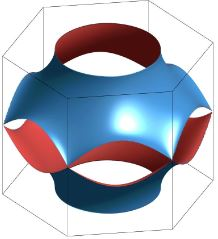Chemical potential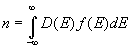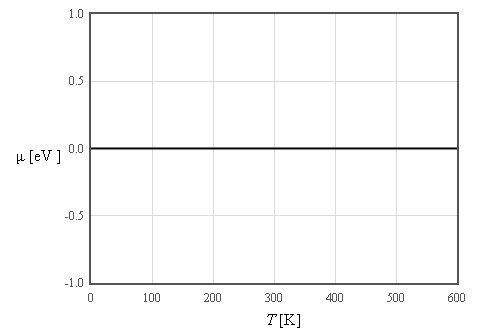The density of states for graphene is symmetric around the Fermi energy so the chemical potential is nearly temperature independent.The density of states for simple cubic is symmetric around the Fermi energy so the chemical potential is nearly temperature independent.

Internal energy distribution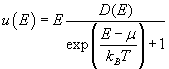Internal energy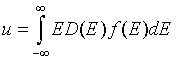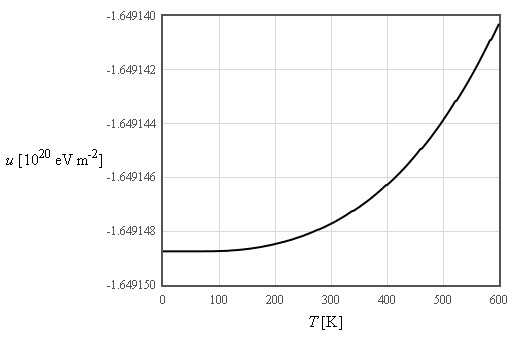The electronic contribution to the internal energy density is small for graphene since the density of states is zero at the Fermi energy.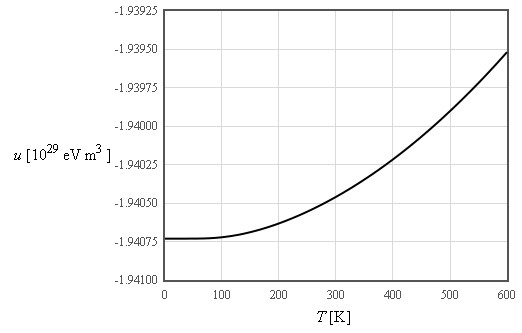Helmholtz free energy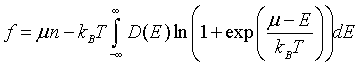Entropy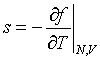Specific heat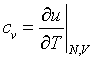The specific heat for graphene is small because the density of states is zero at the Fermi energy.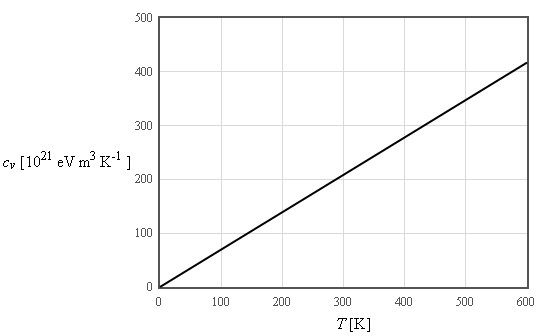Pressure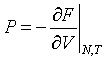Bulk modulus